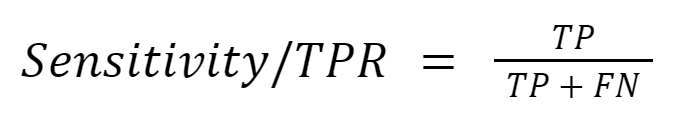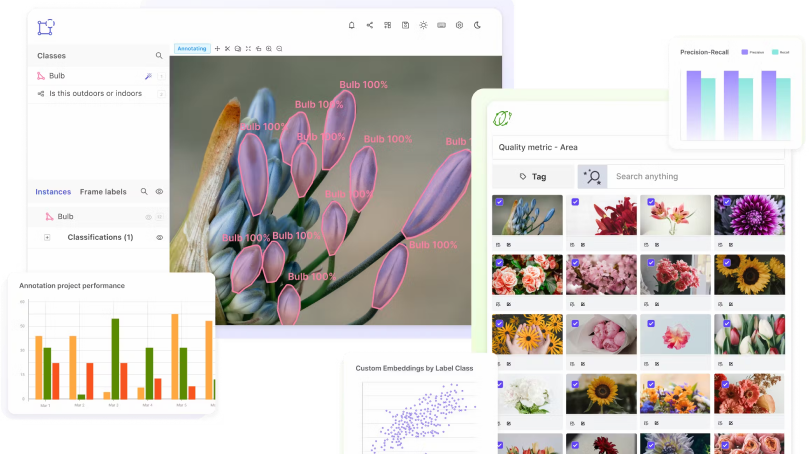# True Positive Rate (TPR)

Encord Computer Vision Glossary

True Positive Rate (TPR), also known as sensitivity, is a metric used to evaluate the performance of binary classification models. It is defined as the ratio of correctly predicted positive instances to the total number of actual positive instances.

In a binary classification problem, a positive instance is one that is classified as positive by the model, while a negative instance is classified as negative. The true positive rate is the proportion of positive instances that are correctly classified by the model.

Mathematically, TPR is expressed as TPR = TP / (TP + FN), where TP is the number of true positive instances, and FN is the number of false negative instances.The true positive rate is an important metric in many fields, including medicine, where it is used to evaluate the performance of diagnostic tests, and machine learning, where it is used to evaluate the performance of binary classification models. A high true positive rate indicates that the model is correctly identifying most of the positive instances, while a low true positive rate indicates that the model is missing many positive instances.

Scale your annotation workflows and power your model performance with data-driven insightsIn practice, the true positive rate is often used in conjunction with other metrics, such as the false positive rate and the area under the ROC curve, to provide a more complete picture of a model's performance#### Discuss this blog on Slack

Join the Encord Developers community to discuss the latest in computer vision, machine learning, and data-centric AI

Join the community
###### Subscribe